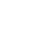# Creating your own length function using recursion in Purescript

Published 4 years ago
Updated 3 years ago

More in-depth information into Partials "Why have a Partial type class?" https://github.com/purescript/documentation/blob/master/guides/The-Partial-type-class.md

Instructor: [00:00] To get started, we'll import a couple of new functions. We'll import data.array, and from there, we'll bring in the null. Then we'll import data array partial, and from there, we'll bring in tail. After that, we'll import partial.unsafe, from there, we'll bring in unsafe partial.

[00:23] Now, let's make our function. We'll call it length, and our type of length is a for all A, array of A. It returns us an int. On a new line, length, and we will call our input R. That equals, if null is an array -- that's checking if the array is null, if the array has nothing in it -- then we'll return zero.

[00:48] Else, we'll do one plus the length, unsafe partial tail R. It should be noted that a proper implementation of length doesn't use unsafe partial. It's not something you really want to do in your code, because what's happening here is, if you do get an error, this will blow up at runtime, rather than compile time.

[01:07] That's living life right on the edge, and we don't want that. We want to handle as many errors at compile time. For now, we'll use this just for an example basis. Time to test it out. We'll do length, and we'll pass it an array of one, two, three, and four.

[01:24] If you look at the results, that returns us four, which is what we expect, because our array has four attributes. Its length is four. Let's clean up this function with a little bit of pattern matching. We'll add length empty array equals zero.

[01:38] This is basically replacing our if, then, and else. If length is empty array, we'll return zero. Otherwise, we'll run what was in the R statement. Now, let me explain what's happening on line 13. We have unsafe partial, I explained, and tail, which we pass in R.

[01:52] A quick example is, if we pass tail the array of one, two, and four, that will return us two and four. Next, I'm going to write out how we're recursively going over the elements in the array, and adding them one by one to give us the answer of the length, which is four.

[02:09] We'd start off by passing in our array -- that would be one -- plus the length of the tail of that array. In our case, that would be two, three, and four. On the next iteration, it would be three and four, and then four.

[02:22] You can see why each at step, we're adding one plus. Once our array is empty, we'll return zero, which is what breaks us out of our recursion. We're left with one plus one, plus one, plus one. Pay special attention to how everything's embedded every time you call length. If this was to happen five times, you'd have a calculation that's embedded five levels deep.

[02:44] There you go. You've created your own length function using recursion.Vasiliy Vanchuk
~ 3 years ago